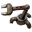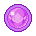## Discrepancy on Damage page on wiki

Anything related to the content on our wiki (https://wiki.factorio.com/)

Moderator: Bilka

JonathanMorgan
Burner InserterPosts: 6
Joined: Sat Apr 22, 2017 3:33 pm
Contact:

### Discrepancy on Damage page on wiki

When looking at the table for Damage, Flat Resistance, Modified damage (with 0% resistance) I've noticed it doesn't line up with the formula when modified damage less than 1.

For example, The table says when raw damage is 5 and Flat Resistance is 5 the modified damage is 1/2 (.5). However, when inserting those values into the following formula:
M=1/(1-(D-R))=1/(1+R-D)
M = 1/(1-(5-5)) = 1/(1+5-5) = 1/1 = 1

The result is 1 not .5

I would think the table is correct for all the examples, not the formula, since it would make sense in the example of:
raw damage is 5 and Flat Resistance is 4 the modified damage is 1. As a result, the damage should decrease not stay the same if flat resistance is 5. But this needs verified.

I think the formula should be:
M=1/(2-(D-R))=1/(2+R-D)

Also, this means that the formula that incorporates the percentile resistance (when modified damage less than 1) needs modified if the assumption above is correct:
F = (1 - P) / (1 + R - D)

Wiki page in question:
https://wiki.factorio.com/Damage

Bilka
Factorio StaffPosts: 2412
Joined: Sat Aug 13, 2016 9:20 am
Contact:

### Re: Discrepancy on Damage page on wiki

You are right, the formula was wrong. I found the right formulas in the source code and corrected the page.
I'm an admin over at https://wiki.factorio.com. Feel free to contact me if there's anything wrong (or right) with it.

JonathanMorgan
Burner InserterPosts: 6
Joined: Sat Apr 22, 2017 3:33 pm
Contact:

### Re: Discrepancy on Damage page on wiki

Thank you very much Bilka.

### Who is online

Users browsing this forum: No registered users